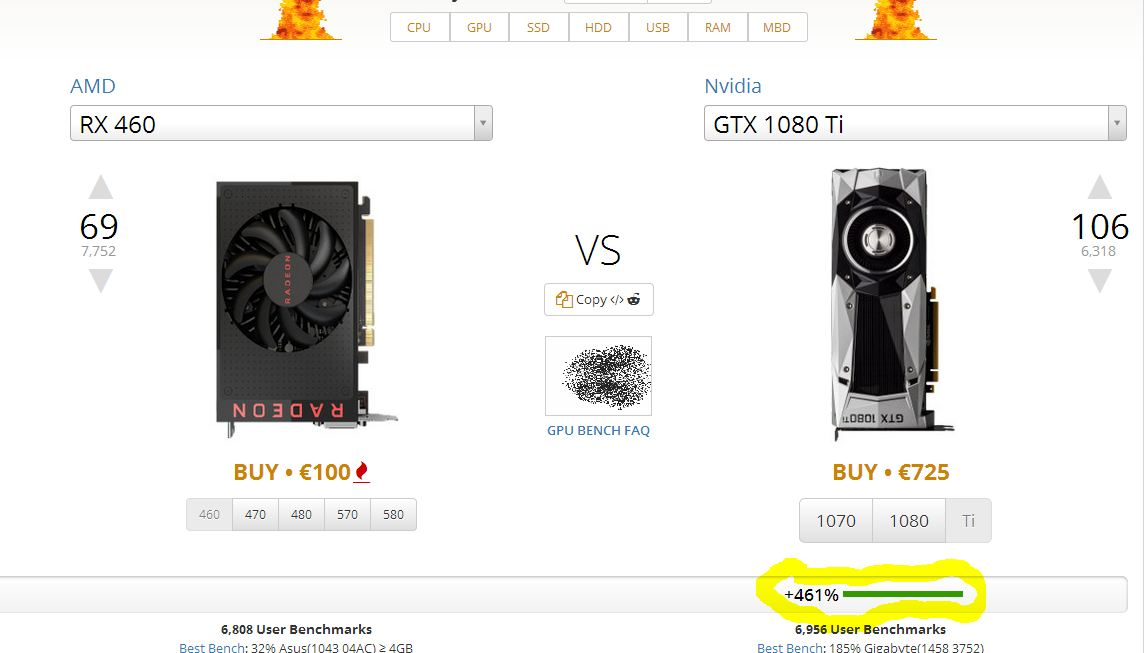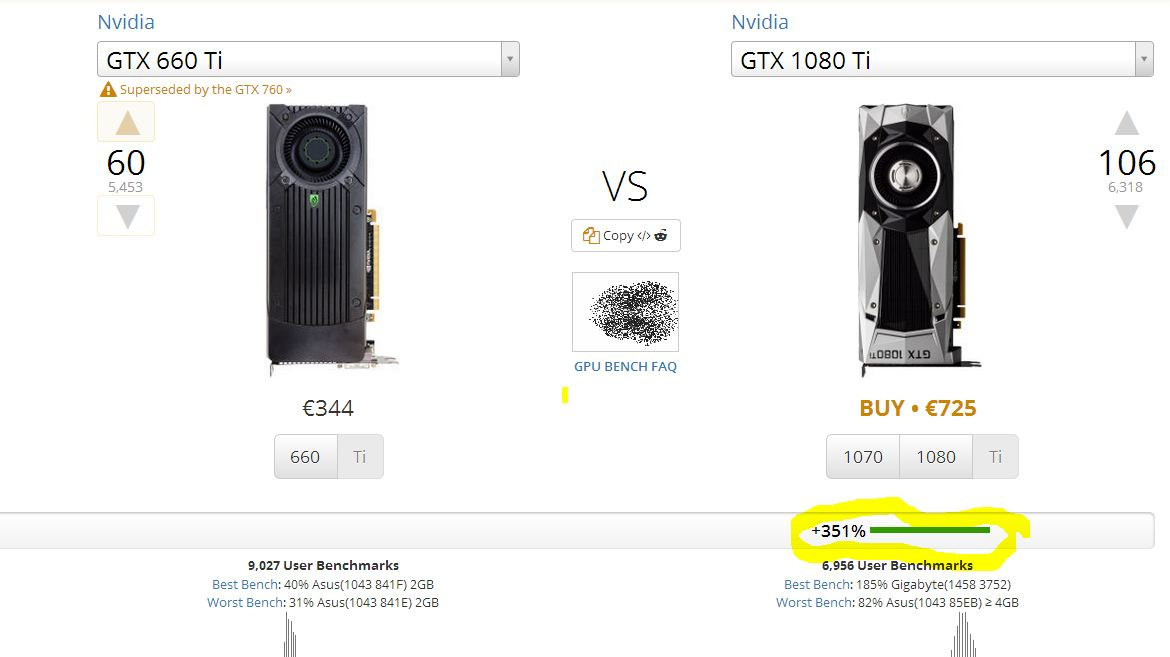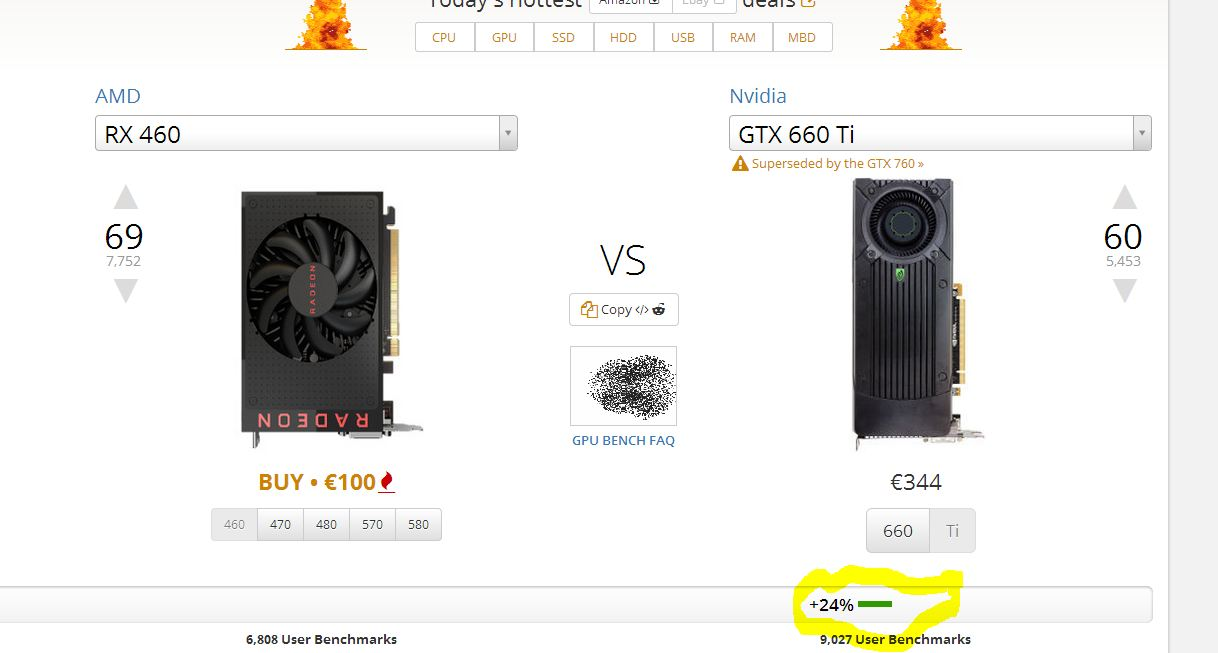#### Peter82p

##### Prominent
I did a test on Userbenchmark.com. The difference should be approximately 100% between the RX 460 and the GTX 660 Ti. Something runs wrong with this website? How does such a result come about? The comparison between the 1080 TI and the 660 TI shows a difference of 351%.
The comparison of the 1080 Ti and the RX 460 shows a difference of 461%.
But the comparison between the 660 TI and the RX 460 shows only a difference of 24%.
How then can the difference of the two upper comparisons show a difference of 110% ?, if the lower comparison says that between the GTX 660 TI and the RX 460 is only a difference of 24%?
Sorry for my bad English, its not my native Language.#### raisonjohn

##### Splendid
When it comes to percentage change, it's not a simple addition/subtraction of the difference between the GTX 660 Ti's speed (compared to a GTX 1080 Ti) and the RX 460's speed (compared to a GTX 1080 Ti). Based on UserBenchmark GPU's effective speed you posted, it shows that:

GTX 1080 Ti has a 351% effective speed compared to a GTX 660 Ti, while the GTX 1080 Ti has a 461% effective speed compared to an RX 460.

Let's see if the UserBenchmark rating is correct when it states that the GTX 660 Ti only has a 24% effective speed compared to an RX 460.

Since both GTX 1080 Ti is compared to a certain card (i.e., the GTX 660 Ti and the RX 460), the baseline or reference of the cards being compared to is set at 100% (or 1), such that,

GTX 1080 Ti = (GTX 660 Ti @ 100%) + 351%
GTX 1080 Ti = (RX 460 @ 100%) + 461%

Where both GTX 660 Ti and RX 460 are set at baseline 100%, it means,

GTX 1080 Ti = 100% + 351% = 451% (or 4.51 times faster) than a GTX 660 Ti
GTX 1080 Ti = 100% + 461% = 561% (or 5.61 times faster) than an RX 460

Now how fast is the GTX 660 Ti compared to the RX 460 as baseline/reference?

Since given the above two equations for GTX 1080 Ti, we see that the GTX 660 Ti multiplied by 4.51, and RX 460 multiplied by 5.61 are the same (both equate to the GTX 1080 Ti speed):

(GTX 660 Ti * 4.51) = (RX 460 * 5.61)

So, transposing the above,

(GTX 660 Ti / RX 460) = (5.61 / 4.51)
(GTX 660 Ti / RX 460) = 1.24 (or 124%)

Solving further the above equation, with respect to RX 460 as 100% baseline speed to be compared to the GTX 660 Ti, we have,

(GTX 660 Ti) = (124%) * (RX 460 @ 100%)
GTX 660 Ti = 1.24 * 1 = 1.24 (or ~124%)

This means that the GTX 660 Ti is 124% (or 1.24 times) faster than an RX 460.

Getting the speed (percentage change), we have,

Speed Change = Speed of GTX 660 Ti - Speed of RX 460
Speed Change = 124% - 100%
Speed Change = 24%

That's the UserBenchmark GPU effective speed rating of the GTX 660 Ti with respect to the RX 460 (at 100% baseline).

#### CRO5513Y

##### Reputable
Probably because you are looking at overall comparison scores combined. The GTX 1080 Ti is leagues ahead because it holds such high percentages over the 660 Ti in every graph where as the RX 460 whilst it loses in Overclocking and Benchmark scores, it wins in other comparisons like value and age if you scroll down you'll see what i mean. The 660 Ti still wins of course but as far as i know the overall score at the top is made up of a relative combination of the sub-categories below it. Hope this helps#### raisonjohn

##### Splendid
When it comes to percentage change, it's not a simple addition/subtraction of the difference between the GTX 660 Ti's speed (compared to a GTX 1080 Ti) and the RX 460's speed (compared to a GTX 1080 Ti). Based on UserBenchmark GPU's effective speed you posted, it shows that:

GTX 1080 Ti has a 351% effective speed compared to a GTX 660 Ti, while the GTX 1080 Ti has a 461% effective speed compared to an RX 460.

Let's see if the UserBenchmark rating is correct when it states that the GTX 660 Ti only has a 24% effective speed compared to an RX 460.

Since both GTX 1080 Ti is compared to a certain card (i.e., the GTX 660 Ti and the RX 460), the baseline or reference of the cards being compared to is set at 100% (or 1), such that,

GTX 1080 Ti = (GTX 660 Ti @ 100%) + 351%
GTX 1080 Ti = (RX 460 @ 100%) + 461%

Where both GTX 660 Ti and RX 460 are set at baseline 100%, it means,

GTX 1080 Ti = 100% + 351% = 451% (or 4.51 times faster) than a GTX 660 Ti
GTX 1080 Ti = 100% + 461% = 561% (or 5.61 times faster) than an RX 460

Now how fast is the GTX 660 Ti compared to the RX 460 as baseline/reference?

Since given the above two equations for GTX 1080 Ti, we see that the GTX 660 Ti multiplied by 4.51, and RX 460 multiplied by 5.61 are the same (both equate to the GTX 1080 Ti speed):

(GTX 660 Ti * 4.51) = (RX 460 * 5.61)

So, transposing the above,

(GTX 660 Ti / RX 460) = (5.61 / 4.51)
(GTX 660 Ti / RX 460) = 1.24 (or 124%)

Solving further the above equation, with respect to RX 460 as 100% baseline speed to be compared to the GTX 660 Ti, we have,

(GTX 660 Ti) = (124%) * (RX 460 @ 100%)
GTX 660 Ti = 1.24 * 1 = 1.24 (or ~124%)

This means that the GTX 660 Ti is 124% (or 1.24 times) faster than an RX 460.

Getting the speed (percentage change), we have,

Speed Change = Speed of GTX 660 Ti - Speed of RX 460
Speed Change = 124% - 100%
Speed Change = 24%

That's the UserBenchmark GPU effective speed rating of the GTX 660 Ti with respect to the RX 460 (at 100% baseline).

#### Peter82p

##### Prominent
Thanks for the quick responses.